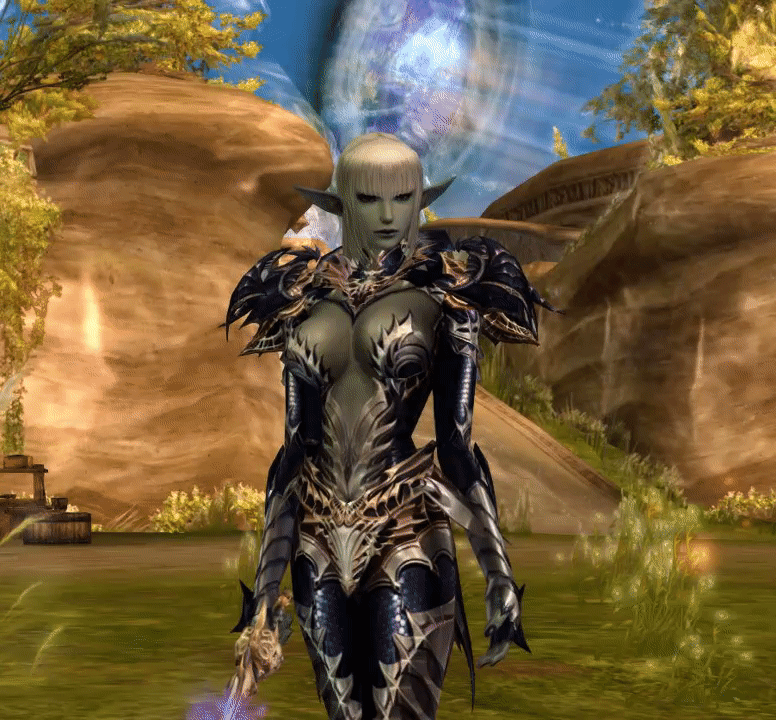# Gildor's Forums

 Pages:Author Topic: .u script TimeTillResetRange / respawn every x second not working?  (Read 350 times)
babyjason
L2tool User
NewbiePosts: 38.u script TimeTillResetRange / respawn every x second not working? « on: January 07, 2021, 12:31 »

Hello, i try to make an effect to automatic respawn every 10 seconds,  meaning :
1. effect shows up , fades out
2. effect automaticaly shows up after 10 second for exemple again
3. repeats the above untill effect is on character

there is function in script:     TimeTillResetRange=(Min=10.0,Max=15.0)  , but the effect shows up in game 1 time and after never again untill effect is manualy cleared and respawned.  My engine has this function ( i checked in engine.u with wotgraill ) .   The only thing that is missing is "        AlwaysFullSpawnRate=true" inside each effect, which i tried to remove also, but the result is the same, i cannot find any info of such function (ALwaysFullSpawnRate), but i read in unrealengine2 documentation that TimeTillRespawnRange should work, so i do not understand why it does not?

Any idea why isn't this feature not working or how could i make an effect re-spawn automaticaly every x seconds?

this is the entire script"« Last Edit: January 07, 2021, 13:01 by babyjason »Logged
babyjason
L2tool User
NewbiePosts: 38Re: .u script TimeTillResetRange / respawn every x second not working? « Reply #1 on: January 07, 2021, 12:32 »

Code:
class ave_brooch extends Emitter;

defaultproperties
{
Begin Object Class=MeshEmitter Name=MeshEmitter21
StaticMesh=StaticMesh'LineageEffectsStaticmeshes.White.white_Protect01'
ColorScale(0)=(RelativeTime=0.0,Color=(B=135,G=224,R=254,A=255))
ColorScale(1)=(RelativeTime=1.0,Color=(B=74,G=158,R=251,A=255))
Opacity=0.28
MaxParticles=1
AlwaysFullSpawnRate=true
Name="glow"
StartSizeRange=(X=(Min=0.2,Max=0.2),Y=(Min=0.2,Max=0.2),Z=(Min=0.2,Max=0.2))
InitialParticlesPerSecond=1000.0
AutomaticInitialSpawning=false
End Object
Emitters(0)=MeshEmitter'MeshEmitter21'
Begin Object Class=VertMeshEmitter Name=VertMeshEmitter16
VertexMesh=VertMesh'LineageEffectMeshes.hero_aura00'
UseMeshBlendMode=false
ColorScale(0)=(RelativeTime=0.0,Color=(B=255,G=255,R=255,A=255))
ColorScale(1)=(RelativeTime=0.296429,Color=(B=183,G=210,R=253,A=255))
ColorScale(2)=(RelativeTime=1.0,Color=(B=136,G=182,R=251,A=255))
ColorMultiplierRange=(X=(Min=0.3,Max=0.3),Y=(Min=0.5,Max=0.5),Z=(Min=1.0,Max=1.0))
Opacity=0.86
AlwaysFullSpawnRate=true
Name="Orbital3"
SpinParticles=true
StartSpinRange=(X=(Min=0.0,Max=1.0),Y=(Min=0.0,Max=1.0),Z=(Min=0.0,Max=1.0))
StartSizeRange=(X=(Min=0.005,Max=0.01),Y=(Min=0.005,Max=0.01),Z=(Min=0.005,Max=0.01))
InitialParticlesPerSecond=5.0
AutomaticInitialSpawning=false
InitialDelayRange=(Min=0.3,Max=0.3)
End Object
Emitters(1)=VertMeshEmitter'VertMeshEmitter16'
Begin Object Class=MeshEmitter Name=MeshEmitter24
StaticMesh=StaticMesh'LineageEffectsStaticmeshes.Monster.wispray01'
UseMeshBlendMode=false
RenderTwoSided=true
UseColorScale=true
ColorScale(0)=(RelativeTime=0.0,Color=(B=255,G=255,R=255,A=255))
ColorScale(1)=(RelativeTime=0.489286,Color=(B=147,G=133,R=108,A=255))
ColorScale(2)=(RelativeTime=1.0,Color=(B=255,G=255,R=255,A=255))
ColorScaleRepeats=15.0
ColorMultiplierRange=(X=(Min=0.8,Max=0.8),Y=(Min=0.75,Max=0.75),Z=(Min=0.7,Max=0.7))
Opacity=0.18
MaxParticles=5
AlwaysFullSpawnRate=true
Name="RayMesh"
SpinParticles=true
SpinCCWorCW=(X=0.5,Y=0.5,Z=0.0)
SpinsPerSecondRange=(X=(Min=0.0,Max=0.02),Y=(Min=0.0,Max=0.02),Z=(Min=0.0,Max=0.02))
StartSpinRange=(X=(Min=0.0,Max=1.0),Y=(Min=0.0,Max=1.0),Z=(Min=0.0,Max=1.0))
UseSizeScale=true
UseRegularSizeScale=false
UniformSize=true
SizeScale(0)=(RelativeTime=0.0,RelativeSize=0.3)
SizeScale(1)=(RelativeTime=0.2,RelativeSize=1.0)
SizeScale(2)=(RelativeTime=1.0,RelativeSize=1.2)
StartSizeRange=(X=(Min=0.012,Max=0.025),Y=(Min=0.012,Max=0.025),Z=(Min=0.012,Max=0.025))
InitialParticlesPerSecond=1000.0
AutomaticInitialSpawning=false
End ObjectLogged
babyjason
L2tool User
NewbiePosts: 38Re: .u script TimeTillResetRange / respawn every x second not working? « Reply #2 on: January 07, 2021, 12:32 »

Code:
[quote] Emitters(2)=MeshEmitter'MeshEmitter24'
Begin Object Class=SpriteEmitter Name=SpriteEmitter42
UseColorScale=true
ColorScale(0)=(RelativeTime=0.0,Color=(B=255,G=255,R=255,A=255))
ColorScale(1)=(RelativeTime=0.55,Color=(B=190,G=190,R=190,A=255))
ColorScale(2)=(RelativeTime=1.0,Color=(B=255,G=255,R=255,A=255))
ColorScaleRepeats=9.0
ColorMultiplierRange=(X=(Min=0.7,Max=1.0),Y=(Min=0.7,Max=1.0),Z=(Min=0.7,Max=1.0))
MaxParticles=40
AlwaysFullSpawnRate=true
Name="Ticks1"
StartLocationRange=(X=(Min=-1.5,Max=1.5),Y=(Min=-1.5,Max=1.5),Z=(Min=-1.5,Max=1.5))
UseRevolution=true
RevolutionsPerSecondRange=(X=(Min=0.0,Max=0.1),Y=(Min=0.0,Max=0.1),Z=(Min=0.0,Max=0.1))
UseSizeScale=true
UseRegularSizeScale=false
UniformSize=true
SizeScale(0)=(RelativeTime=0.0,RelativeSize=1.0)
SizeScale(1)=(RelativeTime=1.0,RelativeSize=0.3)
StartSizeRange=(X=(Min=0.1,Max=0.5),Y=(Min=0.1,Max=0.5),Z=(Min=0.1,Max=0.5))
InitialParticlesPerSecond=17.0
AutomaticInitialSpawning=false
Texture=Texture'LineageEffectsTextures.Particles.fx_m_t0085'
TextureUSubdivisions=2
TextureVSubdivisions=2
UseRandomSubdivision=true
SubdivisionEnd=1
InitialDelayRange=(Min=0.3,Max=0.3)
End Object
Emitters(3)=SpriteEmitter'SpriteEmitter42'
Begin Object Class=SpriteEmitter Name=SpriteEmitter43
ColorScale(0)=(RelativeTime=0.0,Color=(B=255,G=255,R=255,A=255))
ColorScale(1)=(RelativeTime=1.0,Color=(B=255,G=255,R=255,A=255))
MaxParticles=1
AlwaysFullSpawnRate=true
Name="par1"
SpinParticles=true
SpinsPerSecondRange=(X=(Min=0.05,Max=0.05),Y=(Min=0.0,Max=0.0),Z=(Min=0.0,Max=0.0))
StartSpinRange=(X=(Min=-1.0,Max=1.0),Y=(Min=0.0,Max=0.0),Z=(Min=0.0,Max=0.0))
UseRegularSizeScale=false
UniformSize=true
StartSizeRange=(X=(Min=1.0,Max=1.0),Y=(Min=1.0,Max=1.0),Z=(Min=1.0,Max=1.0))
InitialParticlesPerSecond=100.0
AutomaticInitialSpawning=false
Texture=Texture'LineageEffectsTextures.Particles.fx_m_t0085'
TextureUSubdivisions=2
TextureVSubdivisions=2
SubdivisionEnd=1
InitialDelayRange=(Min=0.3,Max=0.3)
End Object
Emitters(4)=SpriteEmitter'SpriteEmitter43'
Begin Object Class=SpriteEmitter Name=SpriteEmitter45
ColorScale(0)=(RelativeTime=0.0,Color=(B=255,G=255,R=255,A=255))
ColorScale(1)=(RelativeTime=1.0,Color=(B=255,G=255,R=255,A=255))
Opacity=0.0
MaxParticles=1
Name="Time"
UniformSize=true
InitialParticlesPerSecond=100.0
AutomaticInitialSpawning=false
End Object
Emitters(5)=SpriteEmitter'SpriteEmitter45'
Begin Object Class=SpriteEmitter Name=SpriteEmitter46
ColorScale(0)=(RelativeTime=0.0,Color=(B=255,G=255,R=255,A=255))
ColorScale(1)=(RelativeTime=1.0,Color=(B=255,G=255,R=255,A=255))
MaxParticles=1
AlwaysFullSpawnRate=true
Name="par2"
SpinParticles=true
SpinsPerSecondRange=(X=(Min=-0.05,Max=-0.05),Y=(Min=0.0,Max=0.0),Z=(Min=0.0,Max=0.0))
StartSpinRange=(X=(Min=-1.0,Max=1.0),Y=(Min=0.0,Max=0.0),Z=(Min=0.0,Max=0.0))
UseSizeScale=true
UseRegularSizeScale=false
UniformSize=true
SizeScale(0)=(RelativeTime=0.0,RelativeSize=2.0)
SizeScale(1)=(RelativeTime=0.27,RelativeSize=0.7)
SizeScale(2)=(RelativeTime=1.0,RelativeSize=1.0)
StartSizeRange=(X=(Min=1.0,Max=1.0),Y=(Min=1.0,Max=1.0),Z=(Min=1.0,Max=1.0))
InitialParticlesPerSecond=100.0
AutomaticInitialSpawning=false
Texture=Texture'LineageEffectsTextures.Particles.fx_m_t0085'
TextureUSubdivisions=2
TextureVSubdivisions=2
SubdivisionEnd=1
End Object
Emitters(6)=SpriteEmitter'SpriteEmitter46'
TimeTillResetRange=(Min=10.0,Max=15.0)
AutoDestroy=false
AutoReset=true
bNoDelete=false
bUnlit=false
Rotation=(Pitch=112,Yaw=0,Roll=0)
DrawScale=0.02
SwayRotationOrig=(Pitch=112,Yaw=0,Roll=0)
}
[/quote]Logged
SGER@fjs
NewbiePosts: 4Re: .u script TimeTillResetRange / respawn every x second not working? « Reply #3 on: January 09, 2021, 03:23 »

False ---> True

https://docs.unrealengine.com/udk/Two/EmittersReference.htmlLogged
babyjason
L2tool User
NewbiePosts: 38Re: .u script TimeTillResetRange / respawn every x second not working? « Reply #4 on: January 10, 2021, 12:36 »

i tried with that also, but then particles show up all the time and never end.

as soon as i add  TimeTillResetRange the effect ends 1 time and done, i think this function is broken.Logged
 Pages: